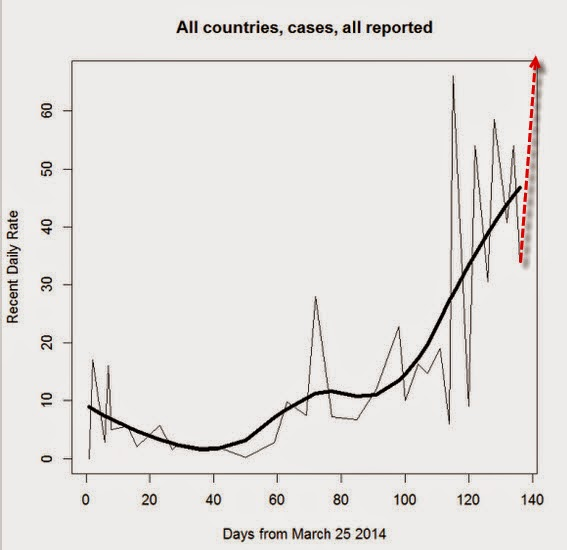## 8/11/14

### " 69 new cases [of Ebola] and 52 new deaths in the last 2 days"; Charts and Math

WHO SAYS 69 NEW EBOLA CASES
REPORTED IN 4 NATIONS AUG. 7-9

Ebola total cases as of 25 March was under 50 total, as opposed to 3 days.http://www.zerohedge.com/news/2014-08-11/french-government-denies-report-several-ebola-cases-outbreak-accelerates-fastest-pac
WHO SAYS 52 DEATHS FROM EBOLA
REPORTED IN 4 NATIONS AUG. 7-9

Ebola total deaths 25 March was 52 total, as opposed to 3 days.

.
.
"Exponential growth occurs when the growth rate of the value of a mathematical function is proportional to the function's current value. Exponential decay occurs in the same way when the growth rate is negative. In the case of a discrete domain of definition with equal intervals it is also called geometric growth or geometric decay (the function values form a geometric progression).

The formula for exponential growth of a variable x at the (positive or negative) growth rate r, as time t goes on in discrete intervals (that is, at integer times 0, 1, 2, 3, ...), is

x_t = x_0(1+r)^t

where x0 is the value of x at time 0. For example, with a growth rate of r = 5% = 0.05, going from any integer value of time to the next integer causes x at the second time to be 1.05 times (i.e., 5% larger than) what it was at the previous time."

http://en.wikipedia.org/wiki/Exponential_growth